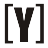# Pounds (lbs) to Kilograms (kg) Conversion

How to Convert Pounds (lbs) to Kilograms (kg): insert number in Pounds box and press convert to conversion into Kilograms (kg).

lb
 lb
oz

: kg
 kg
g
Calculation:

## How to convert lbs to kg

1 lb = 0.45359237 kg

Formula
m (kg) = m (lb) x 0.45359237

The mass m in kilograms (kg) multiplied by 0.45359237

Example: Convert 2 pound to kilogram?
2 lb = 2 kg x 0.45359237 = 0.90718474 kg
2 lb is 0.90718474 kilograms.

Pound to Kilogram conversion table

 Pound [lbs] 0.01 lbs 0.1 lbs 1 lbs 2 lbs 3 lbs 5 lbs 10 lbs 20 lbs 50 lbs 100 lbs 1000 lbs Kilogram [kg] 0.0045359237 kg 0.045359237 kg 0.45359237 kg 0.90718474 kg 1.36077711 kg 2.26796185 kg 4.5359237 kg 9.0718474 kg 22.6796185 kg 45.359237 kg 453.59237 kgYoosFuhl.com Would you like to receive notifications on latest updates? No Yes